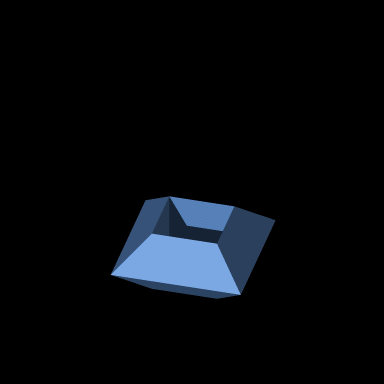Acronym ... Name toroidal tiler© Dihedral angles at h/2-edge between trapezium and trapezium:   arccos(-1/3) = 109.471221° at u-edge between trapezium and trapezium:   90° at x-edge between square and trapezium:   45° Dual combinatorically self-dual

This toroidal polyhedron allows to fill 3D space periodically without gaps and overlaps. (I found that interesting one on Dorozinski's page on space fillings. He is also the originator of the above animation.)

It has a central hole, which is an erased unit cube. The outline is a u-square. The lacing edges are half of the cube's body diagonal, i.e. have the size h/2 = sqrt(3)/2 = 0.866025. Faces are 4 x-squares (sides of the hole) and 8 outer {(t,t,T,T)}-trapeziums. Side lengths thereof clearly are x, h/2, u, h/2. The height of that trapezium thus is q/2 = 1/sqrt(2) = 0.707107. The lower base angles of that trapezium then are t = arccos(1/sqrt(3)) = 54.735610° while the upper base angles are the complement therof, i.e. T = arccos(-1/sqrt(3)) = 125.264390°.

Incidence matrix according to Dynkin symbol

xux4ooo&#(h/2)t   → both heights = 1/2
(hollow x-{4} || pseudo u-{4} || hollow x-{4})

4 * * | 2 1 1 0 0 0 | 2 2 0
* 4 * | 0 1 0 2 1 0 | 2 0 2
* * 4 | 0 0 1 0 1 2 | 0 2 2
------+-------------+------
2 0 0 | 4 * * * * * | 1 1 0  x
1 1 0 | * 4 * * * * | 2 0 0  h/2
1 0 1 | * * 4 * * * | 0 2 0  x
0 2 0 | * * * 4 * * | 1 0 1  u
0 1 1 | * * * * 4 * | 0 0 2  h/2
0 0 2 | * * * * * 4 | 0 1 1  x
------+-------------+------
2 2 0 | 1 2 0 1 0 0 | 4 * *  {(t,t,T,T)}
2 0 2 | 1 0 2 0 0 1 | * 4 *  {4}
0 2 2 | 0 0 0 1 2 1 | * * 4  {(t,t,T,T)}